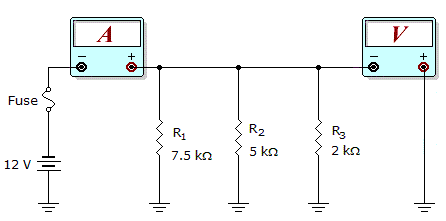# Electronics - Parallel Circuits - Discussion

Discussion Forum : Parallel Circuits - General Questions (Q.No. 11)
11.What would these meter readings indicate about the circuit in the given circuit?

Meter Readings: I = 7.6 mA, V = 12 V

R1 is open.
R2 is open.
The fuse is open.
The circuit is operating normally.
Explanation:
No answer description is available. Let's discuss.
Discussion:
17 comments Page 1 of 2.

CONSIDER THE FOLLOWING:

In Parallel circuit, the current will differ and the voltage will remain same across all the branches. In other words, the voltage across R1, R2 and R3 will be 12V each.

I1= 12/7.5K => 1.6mA
I2= 12/5K => 2.4mA
I3= 12/2K => 6.0mA

In above, the total current 7.6mA will take place correctly when only I1 and I3 present *(since, I1 + I3 = 7.6mA).
Hence, R2 must be discarded/ remove from the circuit. In other words, R2 is open.
(1)

Himanshu Verma said:   1 decade ago
Case1 :When R1 is open,
then the effective resistance R = R2||R3
i.e. 5K||2K = 10/7 K
then current I = V/R = (12*7)/10K = 8.4 mA(Which is not correct ans i.e 7.6 mA)

Case2 : When R2 is open,
then the effective resistance R = R1||R3
i.e. 7.5K||2K = 30/19 K
then current I = V/R = (12*19)/30K = 7.6 mA(Which is d correct ans i.e 7.6 mA)

Case3: When the fuse is open,
then it is not possible to obtain voltage of 12 V

So the correct answer is option [b].

Santosh said:   9 years ago
Voltmeter is in parallel connection, so reading is 12V.

Now for resistance calculation which is connected parallel with 2k ohm resistance (R3):

Equivalent resis. R = 12V/7.6mA = (30/19)K ohm.
So, Rx ll R3 = (30/19)k.
or, (1/Rx)+ (1+R3) = 1/(30/19)k.
or, (1/Rx)+ (1/2) = (19/30).
or, (1/Rx) = (19/30)-(1/2).
or, 1/Rx = 4/30.
or, Rx = 30/4 = 7.5k ohm.

i.e., shows R1 is connected & R2 is opened.

Just look at the fig. above, there is showing batteries positive lead is connected to ground. Not a short to another component which is also grounded. If positive supply connect to the ground else is there current flow in the circuit! Please tell me.

Do the total resistance in the cut by V/I that is 1.578.

Then do the total combination or resist ivory in parallel which resisters combination gives 1.578 ohms and other resistor is open.

Anomie said:   4 years ago
I=7.6mA so.
~7.6=12V/(7.5K*2K/9.5K).
So R2 is not considered for the meter current so it can a open R2.
(1)

Fantastic Himanshu Verma & Saichaitanya ..Great work.

Samir said:   7 years ago
Thank you guys for giving a clear explanation.

Pratiksha said:   7 years ago
Well said, thanks for the given explanation.